## WHY?

Spatial sampling of convolutional neural network is geometrically fixed. This paper suggests two modules for CNN to capture the geometric structure more flexibly.

## WHAT?

Deformable convolution modifies the regular grid $\mathcal{R}$ of convolution network by augmenting $\mathcal{R}$ with offests. offsets are generated by a conv layer with 2N + 1 channels. For example, consider a convolution with 3x3 kernel with dilation 1.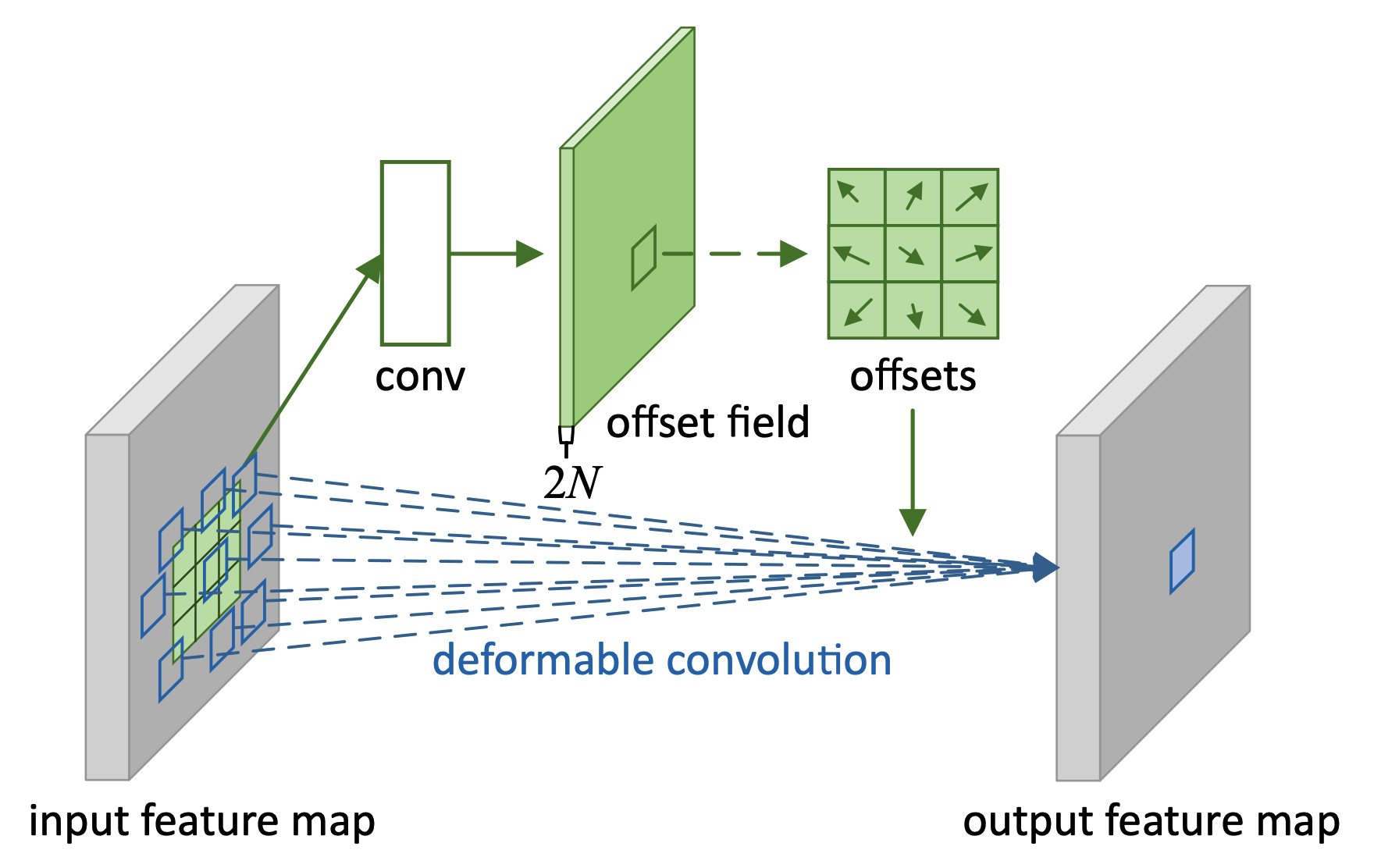Since offsets can be fractional, bilinear interpolation can be used.

This kind of augmentation enable CNN to capture image with various transformation for scale, aspect ratio and rotation.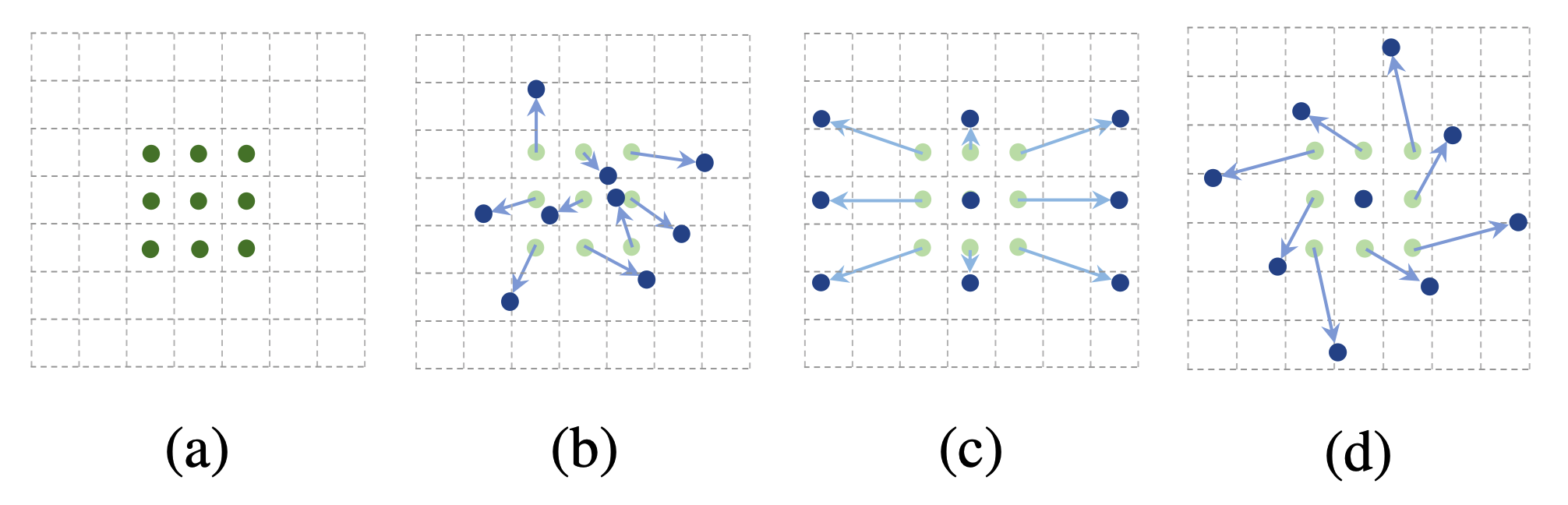Second module is deformable RoI Pooling for image detection. Deformable RoI Pooling divides the RoI into k x k bins and outputs a k x k feature map y. The offsets are generated by a fc layer.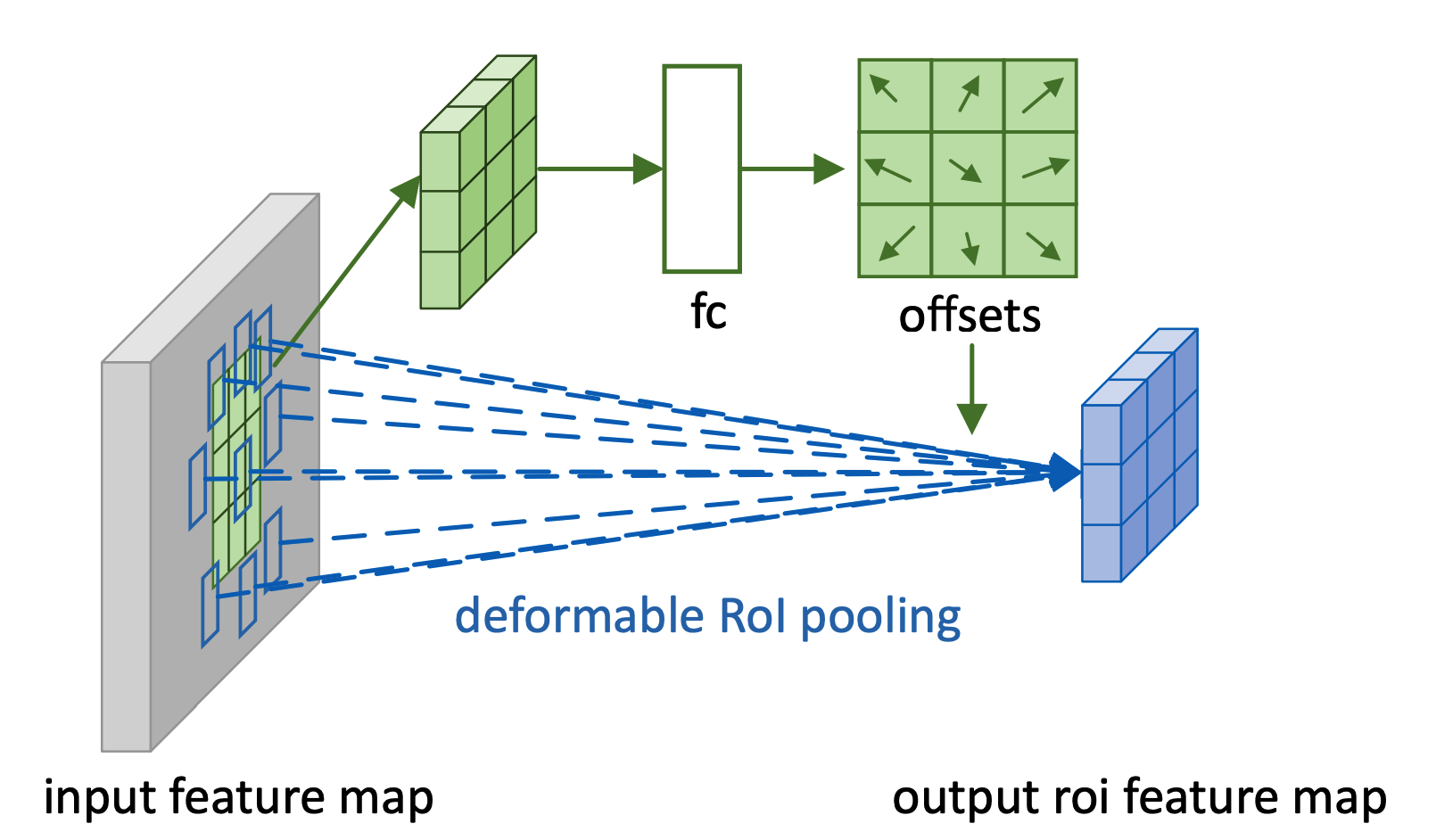Position-Sensitive RoI Pooling replace the general feature map with positive-sensitive score map with $k^2(C+1)$ channels.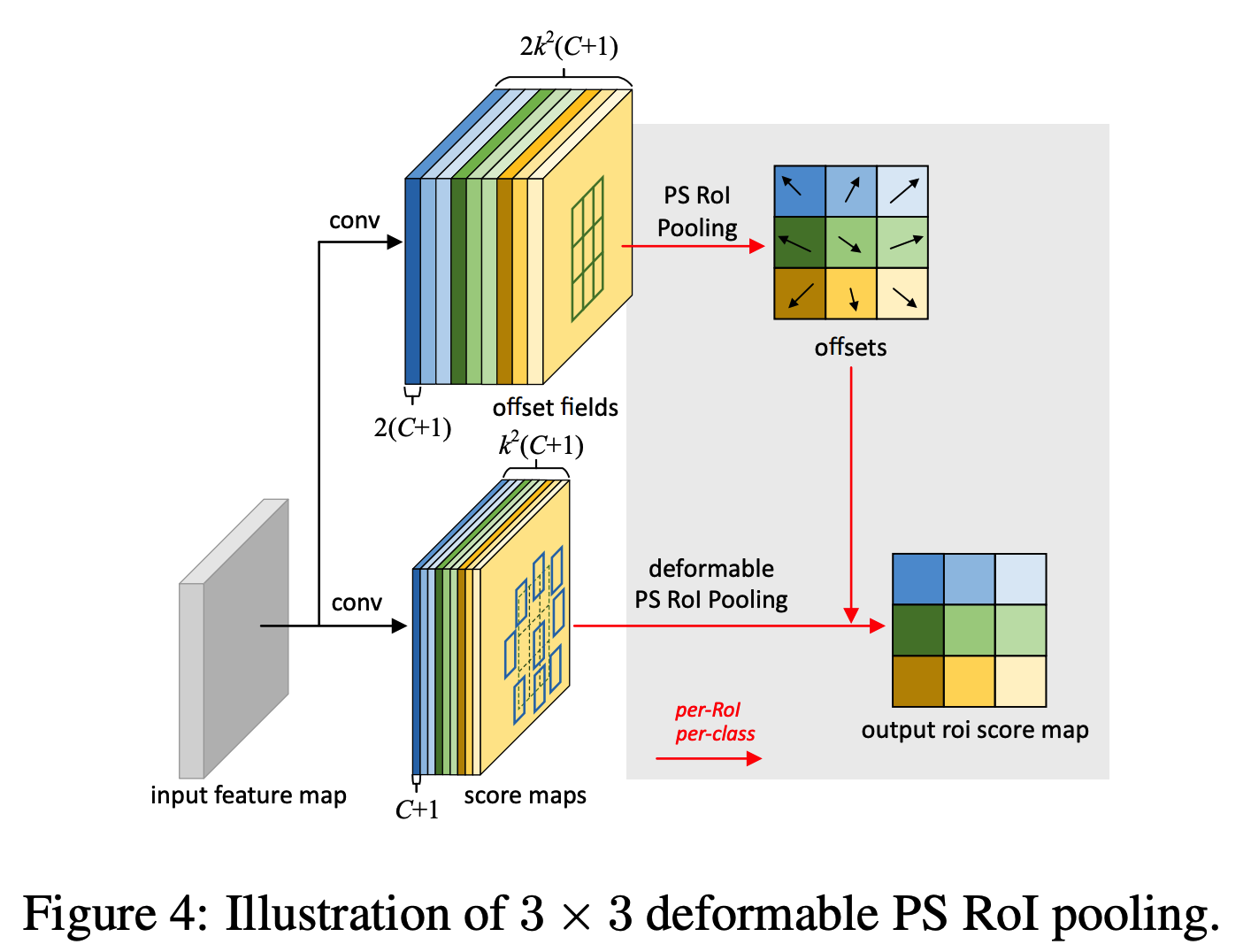## So?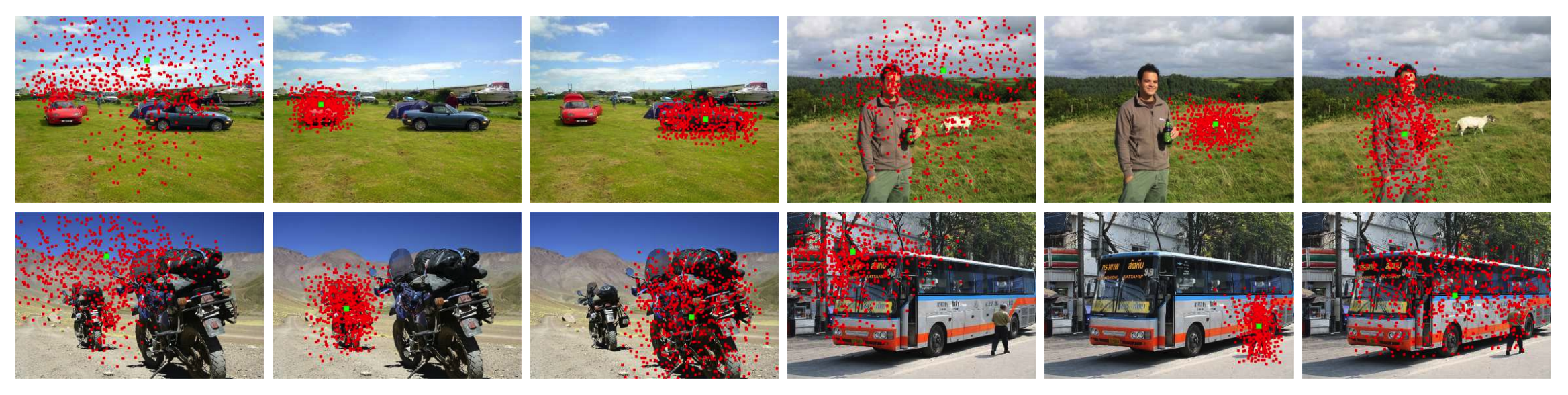Decormable convolution network performed well on semantic segmentation, and object detection than normal convolution network.

## Critic

The amazing proprty of DCN is that the receptive field of its filter can be varied with object size. I assume that feature vectors of DCN may represent real object which can be useful in VQA.

Dai, Jifeng, et al. “Deformable convolutional networks.” CoRR, abs/1703.06211 1.2 (2017): 3.